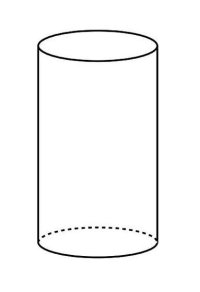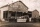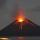# Cylinder - basics

Cylinder with base radius r = 45 dm and height h=22 dm. Calculate:

Result

Area of base A1 =  6361.7 dm2
Cylinder volume V =  139958 dm3
Perimeter of base p =  282.7 dm
Area of shell A2 =  6220.4 dm2
Surface area A3 =  18943.8 dm2

#### Solution:

$A_1 = \pi \cdot 45^2 = 6361.7 \ dm^2$
$V = { A}_1 \cdot 22 = 139958 \ dm^3$
$p = 2\pi \cdot 45 = 282.7 \ \text{ dm }$
$A_2 = p \cdot 22 = 6220.4 \ dm^2$
$A_3 = A _ 2 + 2{ A }_1 = 18943.8 \ dm^2$Our examples were largely sent or created by pupils and students themselves. Therefore, we would be pleased if you could send us any errors you found, spelling mistakes, or rephasing the example. Thank you!

Leave us a comment of this math problem and its solution (i.e. if it is still somewhat unclear...):Be the first to comment!## Next similar math problems:

1. Perimeter of baseThe circumference of the base of the rotating cylinder is same as its height. What is the diameter and height of this cylinder with volume 1 liter?
2. Metal tubeCalculate the metal tube mass 8dm long with the outer radius 5cm and the inner radius 4.5cm and 1cm3 of this metal is 9.5g.
3. Cube into cylinderIf we dip a wooden cube into a barrel with a 40cm radius, the water will rise 10 cm. What is the size of the cube edge?
4. The cylinder 2Find the volume and the lateral area of a cylinder of height 12 inches and a base radius of 4 inches.
5. Collect rain waterThe garden water tank has a cylindrical shape with a diameter of 80 cm and a height of 12 dm. How many liters of water will fit into the tank?
6. Iron densityCalculate the weight of a 2 m long rail pipe with an internal diameter of 10 cm and a wall thickness of 3 mm. The iron density is p = 7.8 g/cm3.
7. 3d printer3D printing ABS filament with diameter 1.75 mm has density 1.04 g/cm3. Find the length of m = 5 kg spool filament. (how to calculate length)
8. Height as diameter of baseThe rotary cylinder has a height equal to the base diameter and the surface of 471 cm2. Calculate the volume of a cylinder.
9. The potThe pot is a cylinder with a volume of V = 7l and an inner diameter of d = 20cm. Find its depth.
10. Ratio of volumesIf the heights of two cylindrical drums are in the ratio 7:8 and their base radii are in the ratio 4:3. What is the ratio of their volumes?
11. Cylinder and its circumferenceIf the height of a cylinder is 4 times its circumference c, what is the volume of the cylinder in terms of its circumference, c?
12. Steel tubeThe steel tube has an inner diameter of 4 cm and an outer diameter of 4.8 cm. The density of the steel is 7800 kg/m3. Calculate its length if it weighs 15 kg.
13. ButterAt the Orville redenbocher popcorn factory there is a tank of artificial butter substitute which is 55 feet tall and 18 feet in diameter. How many gallons of artificial butter substitute can the tank contain?
14. Water flow 2How many litres of water will flow in 7 minutes from a cylindrical pipe 1 cm in diameter, if the water flows at a speed of 30 km per hour
15. The diagram 2The diagram shows a cone with slant height 10.5cm. If the curved surface area of the cone is 115.5 cm2. Calculate correct to 3 significant figures: *Base Radius *Height *Volume of the cone
16. Slant heightThe slant height of cone is 5cm and the radius of its base is 3cm, find the volume of the cone
17. VolcanoThe crater of a volcano is approximately in the shape of a cone of a base 3.1416 sq. Mi. The crater's depth is 1500 ft. How many cubic yards of earth would be required to fill this cavity?